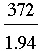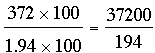SEARCH HOMEMath Central Quandaries & QueriesQuestion from Shelly, a student: How do I divide with a decimal is the DIVISOR ( NOT divisor AND dividend.) ? Also, How do I know when to stop dividing? P.s. please use long divisionShelly,

If you want to divide 372 by 1.94 think of it as a fraction,. Now find an equivalent fraction by multiplying the numerator and denominator by 100.Finally perform long division.

PennyMath Central is supported by the University of Regina and The Pacific Institute for the Mathematical Sciences.